### 5.3. 位操作符

The bitwise operators take operands of integral type. These operators treat their integral operands as a collection of bits, providing operations to test and set individual bits. In addition, these operators may be applied to bitset (Section 3.5, p. 101) operands with the behavior as described here for integral operands.

##### 表 5.3. 位操作符

Operator

Function

Use

~

bitwise NOT（位求反）

~expr

<<

left shift（左移）

expr1 << expr2

>>

right shift（右移）

expr1 >> expr2

&

bitwise AND（位与）

expr1 & expr2

^

bitwise XOR（位异或）

expr1 ^ expr2

|

bitwise OR（位或）

expr1 | expr2

The type of an integer manipulated by the bitwise operators can be either signed or unsigned. If the value is negative, then the way that the "sign bit" is handled in a number of the bitwise operations is machine-dependent. It is, therefore, likely to differ across implementations; programs that work under one implementation may fail under another.Because there are no guarantees for how the sign bit is handled, we strongly recommend using an unsigned type when using an integral value with the bitwise operators. 对于位操作符，由于系统不能确保如何处理其操作数的符号位，所以强烈建议使用unsigned整型操作数。

In the following examples we assume that an unsigned char has 8 bits. The bitwise NOT operator (~) is similar in behavior to the bitset flip (Section 3.5.2, p. 105) operation: It generates a new value with the bits of its operand inverted. Each 1 bit is set to 0; each 0 bit is set to 1:

 unsigned char bits = 0227;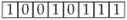bits = ~bits;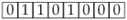The <<, >> operators are the bitwise shift operators. These operators use their right-hand operand to indicate by how many bits to shift. They yield a value that is a copy of the left-hand operand with the bits shifted as directed by the right-hand operand. The bits are shifted left (<<) or right (>>), discarding the bits that are shifted off the end.

<<>> 操作符提供移位操作，其右操作数标志要移动的位数。这两种操作符将其左操作数的各个位向左（<<）或向右（>>）移动若干个位（移动的位数由其右操作数指定），从而产生新的值，并丢弃移出去的位。

 unsigned char bits = 1;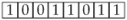bits << 1; // left shift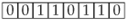bits << 2; // left shift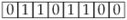bits >> 3; // right shift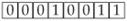The left shift operator (<<) inserts 0-valued bits in from the right. The right shift operator (>>) inserts 0-valued bits in from the left if the operand is unsigned. If the operand is signed, it can either insert copies of the sign bit or insert 0-valued bits; which one it uses is implementation defined. The right-hand operand must not be negative and must be a value that is strictly less than the number of bits in the left-hand operand. Otherwise, the effect of the operation is undefined.

The bitwise AND operator (&) takes two integral operands. For each bit position, the result is 1 if both operands contain 1; otherwise, the result is 0.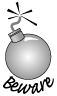It is a common error to confuse the bitwise AND operator (&) with the logical AND operator (&&) (Section 5.2, p. 152). Similarly, it is common to confuse the bitwise OR operator (|) and the logical OR operator(||). 常犯的错误是把位与操作（&）和逻辑与操作（&&）（第 5.2节）混淆了。同样地，位或操作（|）和逻辑或操作（||）也很容易搞混。

Here we illustrate the result of bitwise AND of two unsigned char values, each of which is initialized by an octal literal:

 unsigned char b1 = 0145;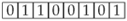unsigned char b2 = 0257;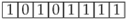unsigned char result = b1 & b2;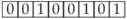The bitwise XOR (exclusive or) operator (^) also takes two integral operands. For each bit position, the result is 1 if either but not both operands contain 1; otherwise, the result is 0.

 result = b1 ^ b2;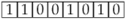The bitwise OR (inclusive or) operator (|) takes two integral operands. For each bit position, the result is 1 if either or both operands contain 1; otherwise, the result is 0.

 result = b1 | b2;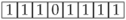#### 5.3.1. bitset 对象或整型值的使用

We said that the bitset class was easier to use than the lower-level bitwise operations on integral values. Let's look at a simple example and show how we might solve a problem using either a bitset or the bitwise operators. Assume that a teacher has 30 students in a class. Each week the class is given a pass/fail quiz. We'll track the results of each quiz using one bit per student to represent the pass or fail grade on a given test. We might represent each quiz in either a bitset or as an integral value:

bitset 类比整型值上的低级位操作更容易使用。观察下面简单的例子，了解如何使用 bitset 类型或者位操作来解决问题。假设某老师带了一个班，班中有 30 个学生，每个星期在班上做一次测验，只有及格和不及格两种测验成绩，对每个学生用一个二进制位来记录一次测试及格或不及格，以方便我们跟踪每次测验的结果，这样就可以用一个bitset对象或整数值来代表一次测验：

```     bitset<30> bitset_quiz1;     //  bitset solution
unsigned long int_quiz1 = 0; // simulated collection of bits
```

In the bitset case we can define bitset_quiz1 to be exactly the size we need. By default each of the bits is set to zero. In the case where we use a built-in type to hold our quiz results, we define int_quiz1 as an unsigned long, meaning that it will have at least 32 bits on any machine. Finally, we explicitly initialize int_quiz1 to ensure that the bits start out with well-defined values.

The teacher must be able to set and test individual bits. For example, assuming that the student represented by position 27 passed, we'd like to be able to set that bit appropriately:

```     bitset_quiz1.set(27);   //  indicate student number 27 passed
int_quiz1 |= 1UL<<27;   //  indicate student number 27 passed
```

In the bitset case we do so directly by passing the bit we want turned on to set. The unsigned long case will take a bit more explanation. The way we'll set a specific bit is to OR our quiz data with another integer that has only one bitthe one we wantturned on. That is, we need an unsigned long where bit 27 is a one and all the other bits are zero. We can obtain such a value by using the left shift operator and the integer constant 1:

```     1UL << 27;  //  generate a value with only bit number 27 set
```

Now when we bitwise OR this value with int_quiz1, all the bits except bit 27 will remain unchanged. That bit will be turned on. We use a compound assignment (Section 1.4.1, p. 13) to OR this value into int_quiz1. This operator, |=, executes in the same way that += does. It is equivalent to the more verbose:

```     //  following assignment is equivalent to int_quiz1 |= 1UL << 27;
int_quiz1 = int_quiz1 | 1UL << 27;
```

Imagine that the teacher reexamined the quiz and discovered that student 27 actually had failed the test. The teacher must now turn off bit 27:

```     bitset_quiz1.reset(27);   // student number 27 failed
int_quiz1 &= ~(1UL<<27);  // student number 27 failed
```

Again, the bitset version is direct. We reset the indicated bit. For the simulated case, we need to do the inverse of what we did to set the bit: This time we'll need an integer that has bit 27 turned off and all the other bits turned on. We'll bitwise AND this value with our quiz data to turn off just that bit. We can obtain a value with all but bit 27 turned on by inverting our previous value. Applying the bitwise NOT to the previous integer will turn on every bit except the 27th. When we bitwise AND this value with int_quiz1, all except bit 27 will remain unchanged.

Finally, we might want to know how the student at position 27 fared. To do so, we could write

```     bool status;
status = bitset_quiz1;       // how did student number 27 do?
status = int_quiz1 & (1UL<<27);  // how did student number 27 do?
```

In the bitset case we can fetch the value directly to determine how that student did. In the unsigned long case, the first step is to set the 27th bit of an integer to 1. The bitwise AND of this value with int_quiz1 evaluates to nonzero if bit 27 of int_quiz1 is also on; otherwise, it evaluates to zero.In general, the library bitset operations are more direct, easier to read, easier to write, and more likely to be used correctly. Moreover, the size of a bitset is not limited by the number of bits in an unsigned. Ordinarily bitset should be used in preference to lower-level direct bit manipulation of integral values. 一般而言，标准库提供的 bitset 操作更直接、更容易阅读和书写、正确使用的可能性更高。而且，bitset 对象的大小不受 unsigned 数的位数限制。通常来说，bitset 优于整型数据的低级直接位操作。

## Exercises Section 5.3.1

 Exercise 5.9: Assume the following two definitions: 假设有下面两个定义 ``` unsigned long ul1 = 3, ul2 = 7; ``` What is the result of each of the following expressions? 下列表达式的结果是什么？ ``` (a) ul1 & ul2 (c) ul1 | ul2 (b) ul1 && ul2 (d) ul1 || ul2 ``` Exercise 5.10: Rewrite the bitset expressions that set and reset the quiz results using a subscript operator. 重写 bitset 表达式：使用下标操作符对测验结果进行置位（置 1）和复位（置 0）。

#### 5.3.2. 将移位操作符用于IO

The IO library redefines the bitwise >> and << operators to do input and output. Even though many programmers never need to use the bitwise operators directly, most programs do make extensive use of the overloaded versions of these operators for IO. When we use an overloaded operator, it has the same precedence and associativity as is defined for the built-in version of the operator. Therefore, programmers need to understand the precedence and associativity of these operators even if they never use them with their built-in meaning as the shift operators.

##### IO 操作符为左结合

Like the other binary operators, the shift operators are left associative. These operators group from left to right, which accounts for the fact that we can concatenate input and output operations into a single statement:

```     cout << "hi" << " there" << endl;
```

executes as:

```     ( (cout << "hi") << " there" ) << endl;
```

In this statement, the operand "hi" is grouped with the first << symbol. Its result is grouped with the second, and then that result is grouped to the third.

The shift operators have midlevel precedence: lower precedence than the arithmetic operators but higher than the relational, assignment, or conditional operators. These relative precedence levels affect how we write IO expressions involving operands that use operators with lower precedence. We often need to use parentheses to force the right grouping:

```     cout << 42 + 10;   // ok, + has higher precedence, so the sum is printed
cout << (10 < 42); // ok: parentheses force intended grouping; prints 1
cout << 10 < 42;   // error: attempt to compare cout to 42!
```

The second cout is interpreted as

```     (cout << 10) < 42;
```

this expression says to "write 10 onto cout and then compare the result of that operation (e.g., cout) to 42."